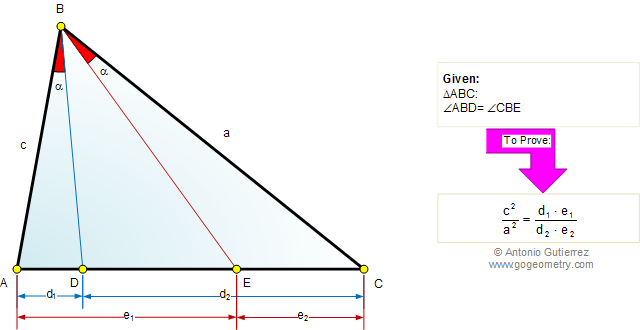# Geometry Problem 595: Triangle, Equal Angles, Isogonal Lines, Similarity

The figure shows a triangle ABC with the angle ABD equal to angle CBE. If AB = c, BC = a, AD = d1, DC = d2, AE = e1, and EC = e2, prove that.
BD and BE are called isogonal conjugates, since they form the same angle with the bisector of angle ABC.Home | Geometry | Search | Problems | All Problems | 591-600 | Triangles | Isogonal Lines | Email | Post a comment or solution | By Antonio Gutierrez